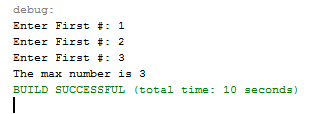# How to Check the Highest Number Value using Java

0
234

This tutorial is all about how to check the highest number value using java between the three different user inputs. This check the highest number value using java  comes with the algorithm that is very useful in a Java program by using an “If function” or a “Switch” function.

It is important that you understand on how to use a Scanner so that the program depends on user input. Also using “If function” to compare different and many conditions and understand on declaring and initializing different variables.

### Check the highest number value in Java Steps

1.Create a new project using your Netbeans.2.Insert the code above your class.

[java]import java.util.Scanner;[/java]

[java]public static void main(String args[]){

}[/java]

4.Declare four variables, one for scanner and 3 for integer data type.

[java]int n_first,n_second,n_third;
Scanner sc = new Scanner(System.in);[/java]
1. Insert the following code after step 4. This statement will ask the user inputs.
[java]System.out.print(“Enter First #: “);
n_first = sc.nextInt();
System.out.print(“Enter First #: “);
n_second = sc.nextInt();
System.out.print(“Enter First #: “);
n_third = sc.nextInt();[/java]

6. Insert the following code after step 5. The code is using nested if statement, assigned different condition, initialized the algorithm, and display output.

[java]if(n_first > n_second){
if (n_first > n_second){
System.out.println(“The max number is ” + n_first);
}else{
System.out.println(“The max number is ” + n_second);
}
}else{
if (n_second > n_third){
System.out.println(“The max number is ” + n_second);
}else{
System.out.println(“The max number is ” + n_third);
}
}[/java]

7. Run the program and the output should look like the image below.8. Complete Source Code

[java]import java.util.Scanner;
public class CheckHighestValue {
public static void main(String args[]){
int n_first,n_second,n_third;
Scanner sc = new Scanner(System.in);

System.out.print(“Enter First #: “);
n_first = sc.nextInt();
System.out.print(“Enter First #: “);
n_second = sc.nextInt();
System.out.print(“Enter First #: “);
n_third = sc.nextInt();

if(n_first > n_second){
if (n_first > n_second){
System.out.println(“The max number is ” + n_first);
}else{
System.out.println(“The max number is ” + n_second);
}
}else{
if (n_second > n_third){
System.out.println(“The max number is ” + n_second);
}else{
System.out.println(“The max number is ” + n_third);
}
}
}
}[/java]

After completing this tutorial, you learn about “nested if statement” and use “multiple conditions”. A nested if statement is an if statement inside an if statement.